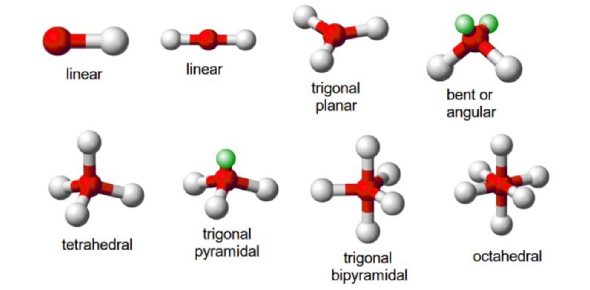# Chemistry Quiz: Molecular Shapes

10 Questions | Total Attempts: 4700SettingsIn the following quiz, on the scientific topic of chemistry, we’re going to be taking our microscopes and focusing in on some of the more intriguing little things you’ll come across in the study; molecules! We’ll be taking a look at the shapes of some molecules and asking you to define some of the particular characteristics of each one. Think you can get them all right? Take the quiz now!

• 1.
Which of the following will water have?A.         B.         C.
• A.

A

• B.

B

• C.

C

• 2.
Which of the following will CO2 have?A.         B.         C.
• A.

A

• B.

B

• C.

C

• 3.
Which of the following will NH3 have?A.         B.         C.
• A.

A

• B.

B

• C.

C

• 4.
Which of the following will methane have?A.         D.                  F.
• A.

A

• B.

D

• C.

F

• 5.
Which of the following is a TETRAHEDRAL?A.         B.         C. D.               E.           F.
• A.

A

• B.

B

• C.

C

• D.

D

• E.

E

• F.

F

• 6.
Which of the following is LINEAR TRIATOMIC?A.         B.         C. D.               E.           F.
• A.

A

• B.

B

• C.

C

• D.

D

• E.

E

• F.

F

• 7.
Which of the following has a 90* angle between atoms?A.         B.         C. D.               E.           F.
• A.

A

• B.

B

• C.

C

• D.

D

• E.

E

• F.

F

• 8.
Which of the following have a 109.5* angle between atoms?A.         B.         C. D.               E.           F.
• A.

A

• B.

B

• C.

C

• D.

D

• E.

E

• F.

F

• 9.
Which of the following is true about C.? Check all the boxes that apply.A.         B.         C. D.               E.           F.
• A.

Its angle is 180*

• B.

CO2, H2O, and Na2S are examples of this

• C.

It involves 3 atoms

• D.

Its called linear tiatomic

• E.

This shape says the molecule is polar

• 10.
All of the following are correct:A.- pyramidal     B.-bent triatomicC.-linear triatomicD.-trigonal planarE.-tetrahedralF.-trigonal bipyramidal
• A.

True

• B.

False

Related TopicsBack to top